# Inequalities and number lines

Inequalities can be represented on a number line.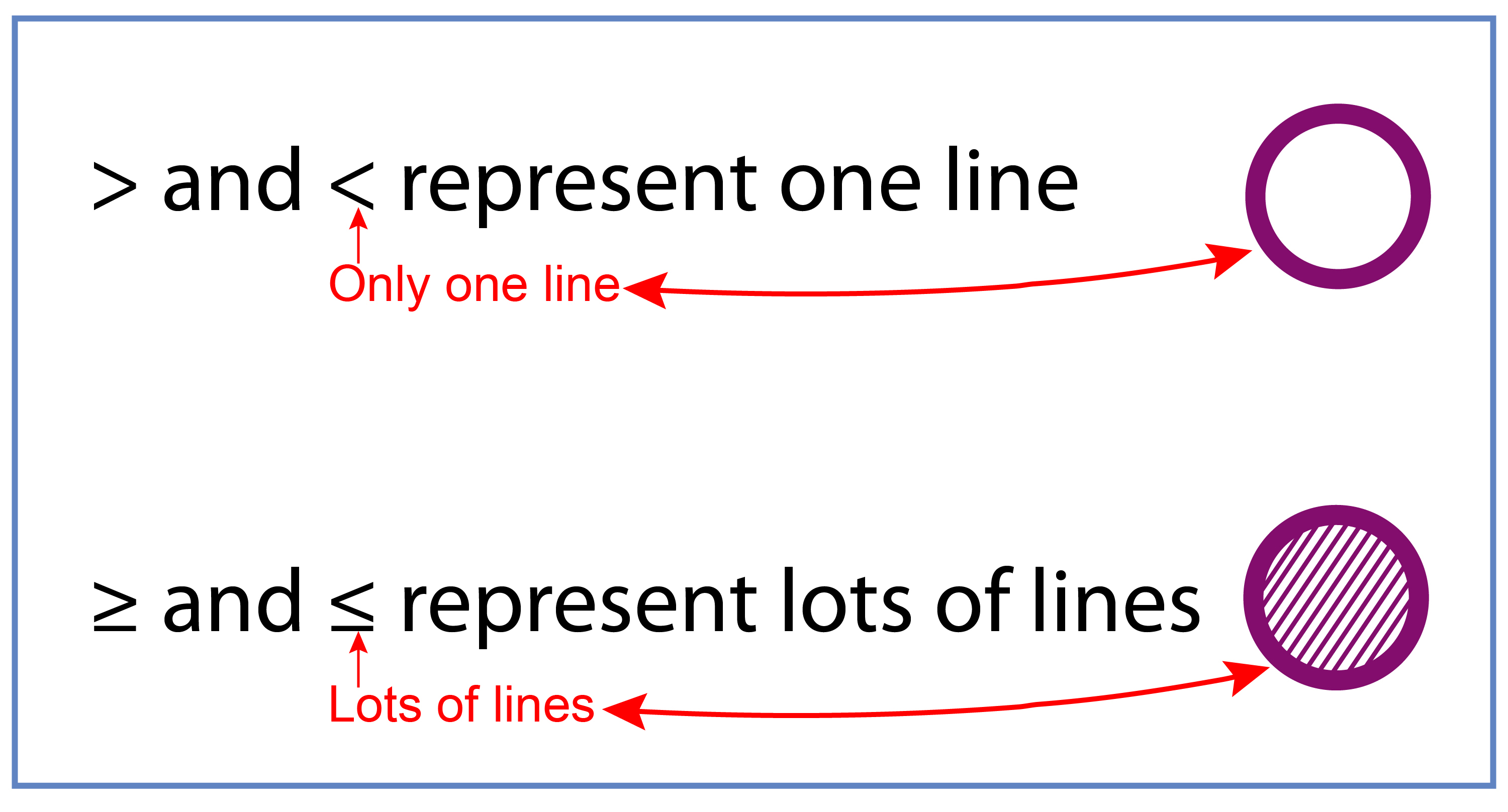And as we have already shown, inequalities can be represented by arrows too: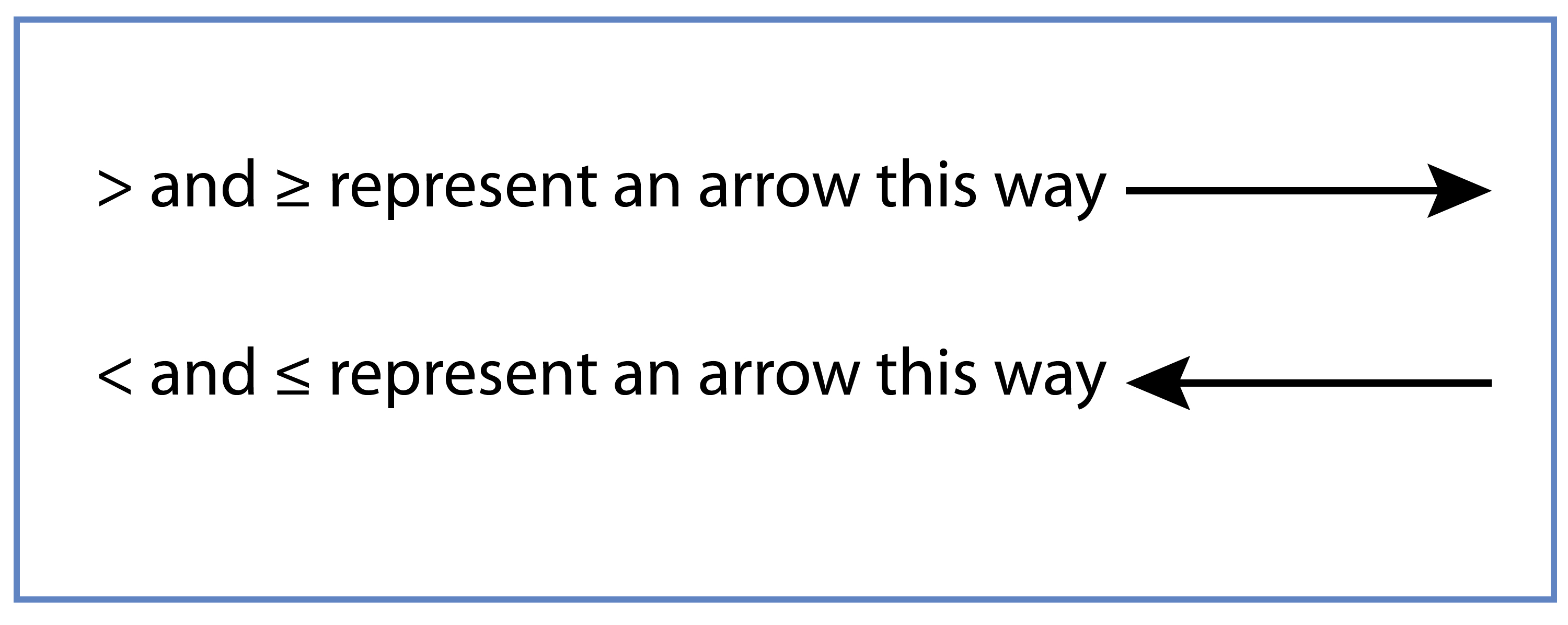And a number line can ONLY mean a line with a number on it.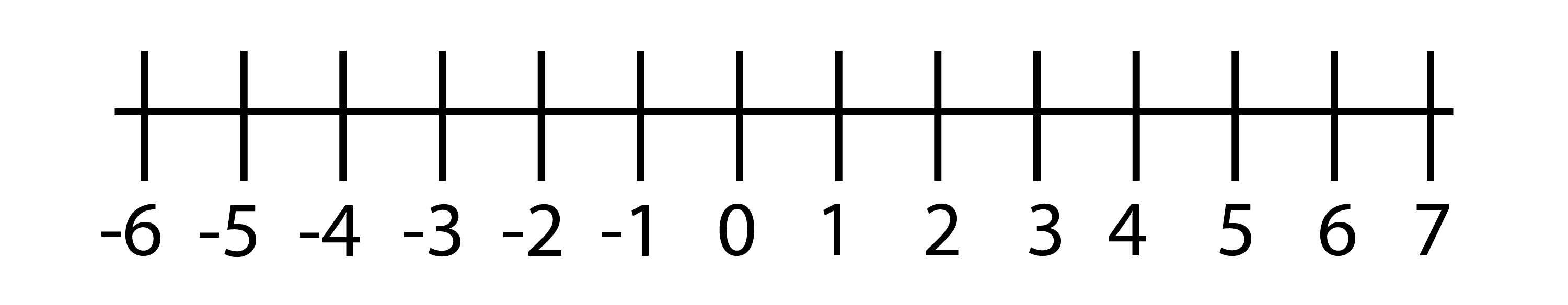And to represent an equality equation on a number line read what the inequality expression says.

Examples

1. Represent x=2 on a number line

This reads x=2 ONLY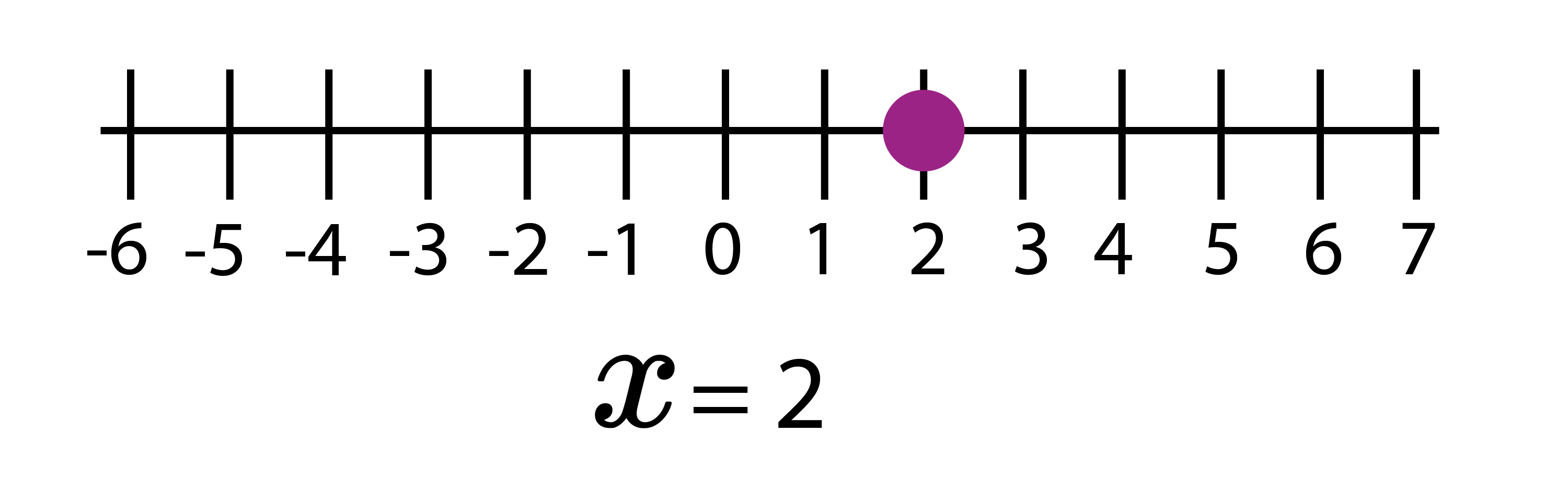2.  On a number line represent

x>=2

This reads as x  is greater than OR equal to 2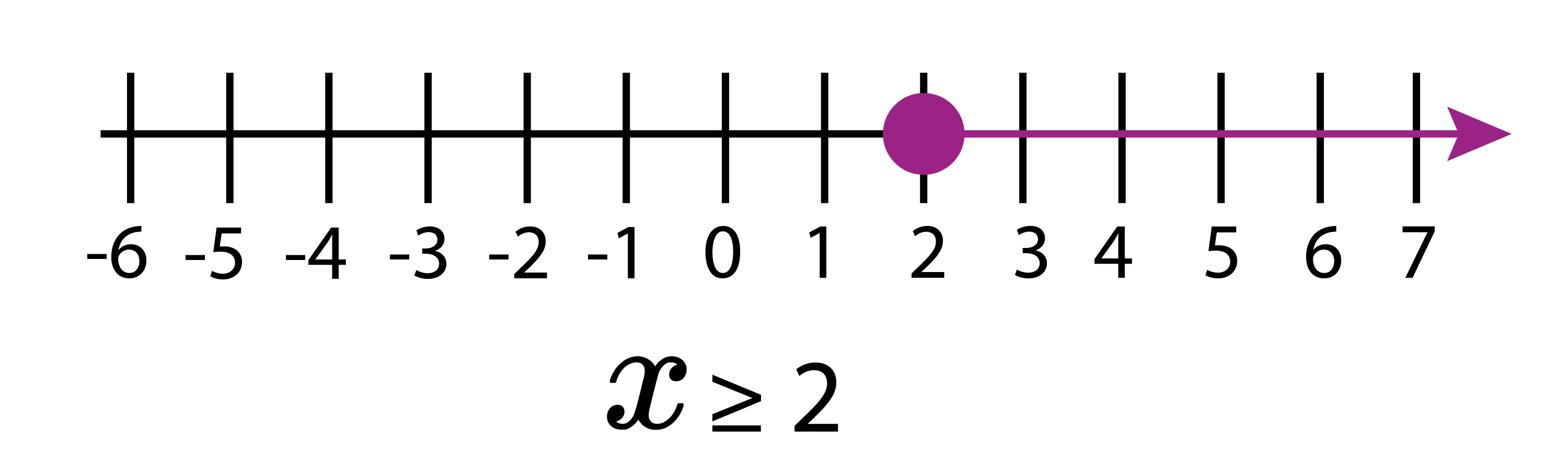3.  On a number line represent

x<=2

This reads as x is less than OR equal to 2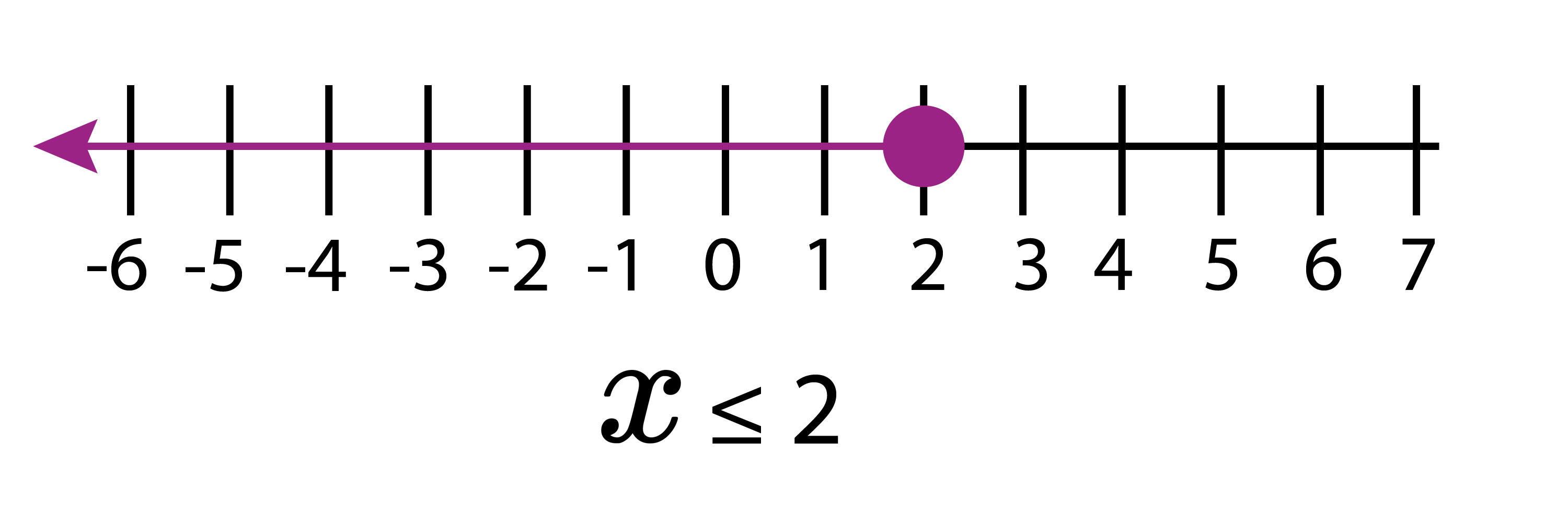4.  On a number line represent

x>2

This reads x is greater than 2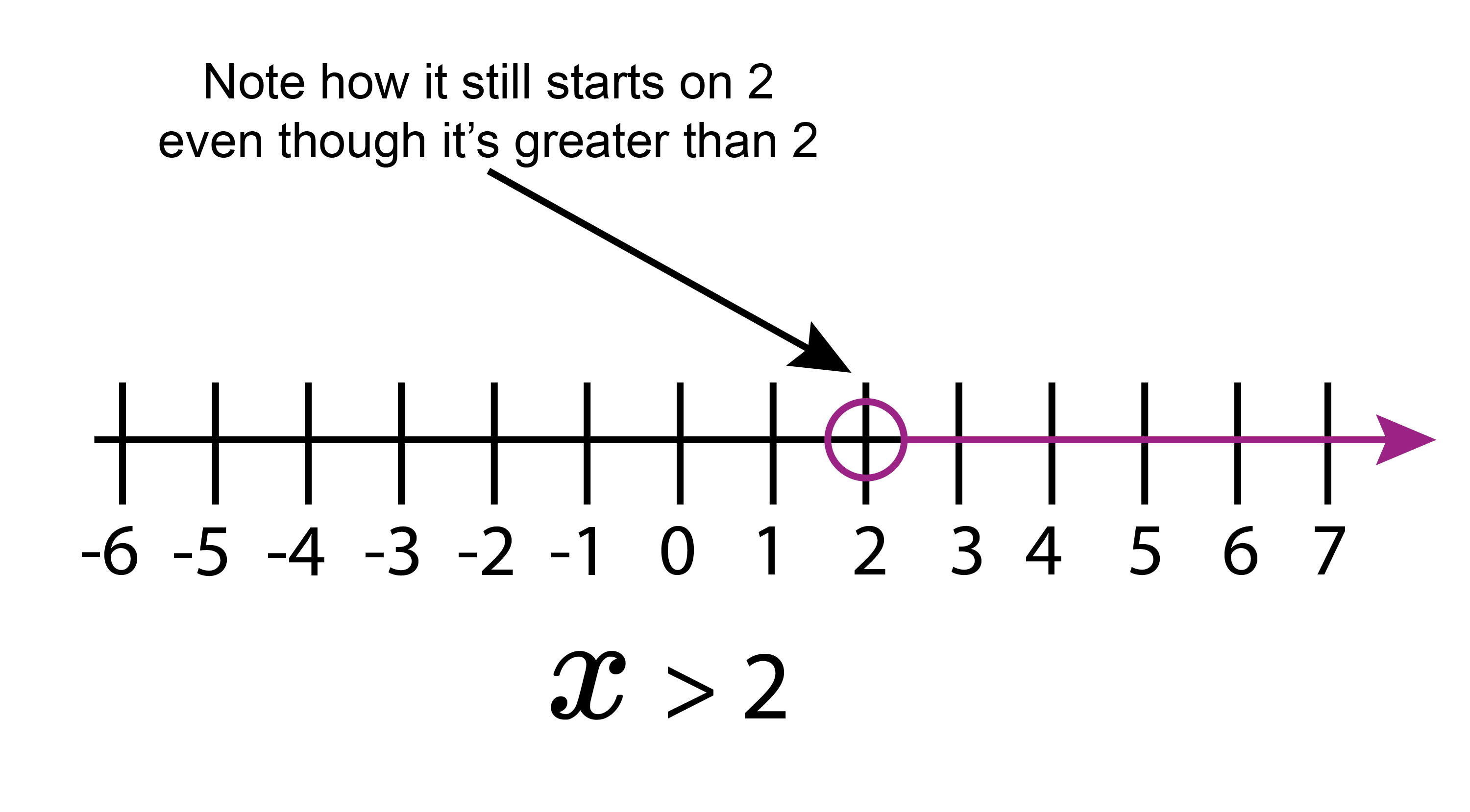5.  On a number line represent

x<2

This reads x is less than 2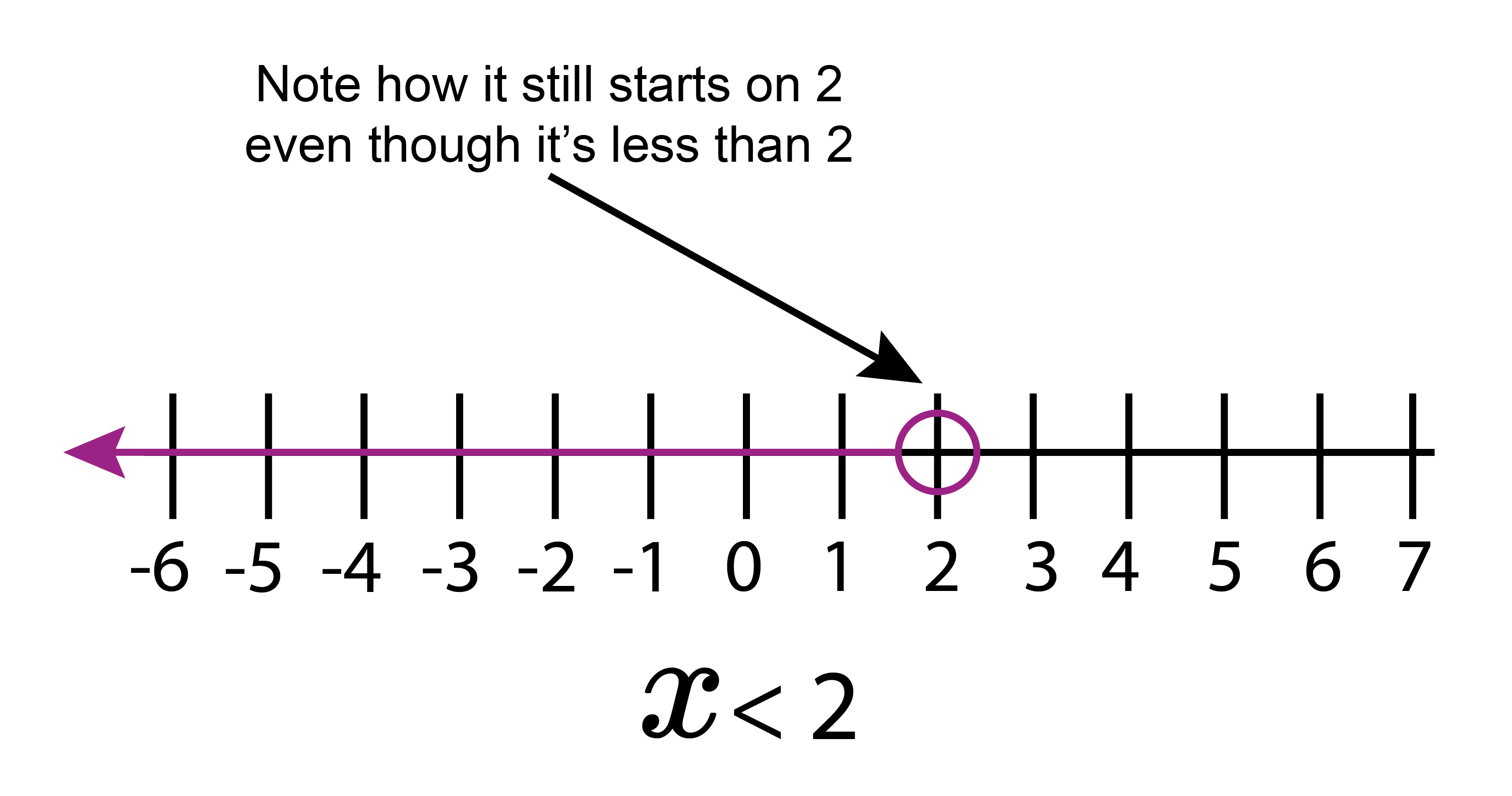Using the above examples will allow you to work out all future problems.

6.  Write down the inequality shown on the number line below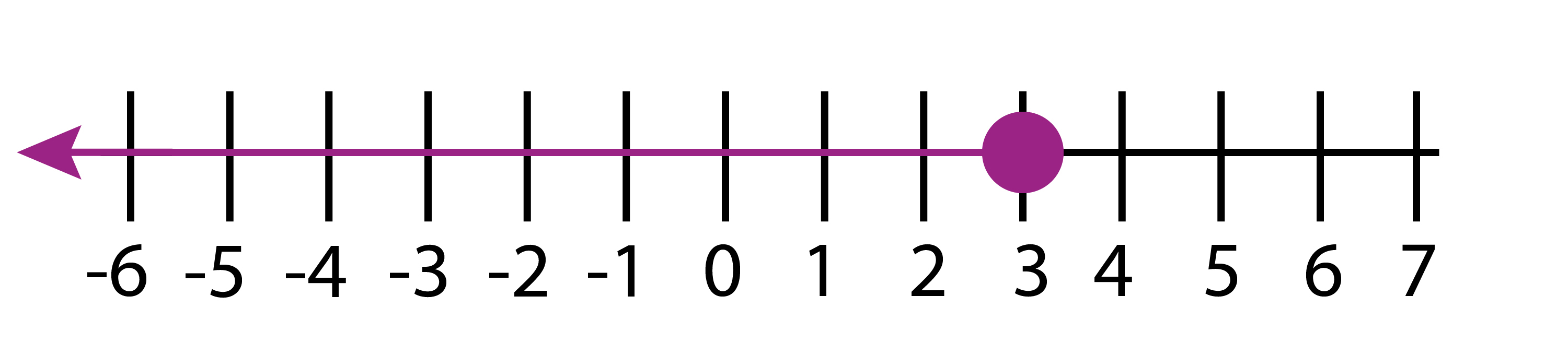The circle above the 3 shows x is less than or equal to 3

x<=3